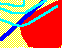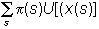7.3 THE ST. PETERSBURG PARADOX

Daniel Bernoulli in 1738 presented a classic paper to the Imperial Academy of Sciences in St. Petersburg that has become known as "The St. Petersburg Paradox." The problem presented is as follows:

"Peter tosses a coin and continues to do so until it should land "heads" when it comes to the ground. He agrees to give Paul one ducat if he gets 'heads' on the very first throw, two ducats if he gets it on the second, four if on the third, eight if on the fourth, and so on, so that with each additional throw the number of ducats he must pay is doubled. Suppose we seek to determine the value of Paul's expectation." (Daniel Bernoulli, translated in Econometrica, XXII (1954) "Exposition of a New Theory of the Measurement of Risk," pp23-36).

The expected payoff can be computed as the sum of the payments weighted by their respective probabilities of occurrence:

1/2 + 2/4 + 4/8 + 8/16 + ...

For any finite n number of throws, the series sum equals n/2 but as n approaches infinity, the sum becomes infinite. Therefore, mathematical expectation implies that Paul should pay an infinite price to acquire this risky opportunity. This mathematical solution is rarely accepted as representing a "reasonable solution" to this problem even though the stakes multiply rapidly with any long sequence of tails.

Earlier independent attempts to resolve this paradox, by Cramer and by Bernoulli himself, invoked preference concepts for money. For example, Cramer's resolution assumes that the "utility" for money is less than the amount of money held. Bernoulli's solution was a variant of this, and assumes that the marginal utility of money is inversely proportional to current wealth.

This example is clearly relevant to the theory of finance. It asks how much an investor is willing to pay for a risky asset. In fact, every investment decision can be reduced to the following steps:

1. identifying a set of investment opportunities,

2. estimating the magnitude, timing, and uncertainty of cash flows for each opportunity,

3. estimating the appropriate opportunity cost of each opportunity, and

4. valuing each opportunity in accordance with individual risk preferences.

The St. Petersburg Paradox is a particular example of valuing a risky investment opportunity.

One solution to the problem is provided by expected utility theory. This theory states that if x(s) is the payoff in event s and p(s) is the probability of event s, then the "value" to the investor isU is called a utility function. In the case of the St. Petersburg paradox, s is a particular infinite realization of heads and tails, and x(s) is the payoff from that sequence. The paradox arises when U[x(s)] = x(s), in which case the "value" is simply equal to the expected value. However, if U is increasing at a decreasing rate (technically, U is a bounded function), then the expected utility is finite.

If we are satisfied with the assumption that expected utility is the correct procedure to use, we can proceed without further development. Alternatively, we can start by assuming that individuals have preferences over risky outcomes, and then investigate conditions under which these preferences can be represented in the form of an expected utility function.

The expected utility theorem establishes that if individual choice behavior conforms to the axioms provided in Appendix A to Chapter 7, then there exists a utility function, U, such that for any two risky investment opportunities L1 and L2, L1 is preferred to L2 if the expected utility from L1 is greater than that from L2.

The expected utility theorem is derived in Appendix A: Expected Utility Theorem. It is assumed that an investor has well-defined preferences over both the set of binary (i.e., two possible values) investment opportunities and the set of all possible values from any investment opportunity. We denote a binary investment opportunity as {p , \$x , \$y }. We call this a triple, where p is the probability of the outcome \$x and (1-p) is the probability of \$y. The expected utility from any binary investment opportunity is defined as:

E(U({p,\$x,\$y})) = pU(\$x) + (1-p)U(\$y)

The utility function U is not unique for any individual, because multiplying U by 10 will also preserve preference rankings. For a utility function, U, we can find different investment opportunities that provide the same level of expected utility. This is developed in the next topic, Investor Risk Preferences.

previous topic

next topic